# JP3927953B2 - Amplitude conversion circuit - Google Patents

## Info

Publication number
JP3927953B2
JP3927953B2 JP2003572178A JP2003572178A JP3927953B2 JP 3927953 B2 JP3927953 B2 JP 3927953B2 JP 2003572178 A JP2003572178 A JP 2003572178A JP 2003572178 A JP2003572178 A JP 2003572178A JP 3927953 B2 JP3927953 B2 JP 3927953B2
Authority
JP
Japan
Prior art keywords
electrode
transistor
voltage
signal
node
Prior art date
Legal status (The legal status is an assumption and is not a legal conclusion. Google has not performed a legal analysis and makes no representation as to the accuracy of the status listed.)
Expired - Fee Related
Application number
JP2003572178A
Other languages
Japanese (ja)
Other versions
JPWO2003073617A1 (en
Inventor

Original Assignee

Priority date (The priority date is an assumption and is not a legal conclusion. Google has not performed a legal analysis and makes no representation as to the accuracy of the date listed.)
Filing date
Publication date
Application filed by 三菱電機株式会社 filed Critical 三菱電機株式会社
Priority to PCT/JP2002/001760 priority Critical patent/WO2003073617A1/en
Publication of JPWO2003073617A1 publication Critical patent/JPWO2003073617A1/en
Application granted granted Critical
Publication of JP3927953B2 publication Critical patent/JP3927953B2/en
Expired - Fee Related legal-status Critical Current
Anticipated expiration legal-status Critical

• 238000006243 chemical reactions Methods 0.000 title claims description 30
• 239000003990 capacitor Substances 0.000 claims description 53
• 230000000295 complement Effects 0.000 claims description 24
• 230000003334 potential Effects 0.000 claims description 7
• 239000010409 thin films Substances 0.000 claims description 3
• 238000010586 diagrams Methods 0.000 description 24
• 239000004973 liquid crystal related substances Substances 0.000 description 13
• 230000004048 modification Effects 0.000 description 8
• 238000006011 modification reactions Methods 0.000 description 8
• 230000000694 effects Effects 0.000 description 5
• 230000003071 parasitic Effects 0.000 description 4
• 230000001629 suppression Effects 0.000 description 2
• 230000015556 catabolic process Effects 0.000 description 1
• 230000001413 cellular Effects 0.000 description 1
• 229910021420 polycrystalline silicon Inorganic materials 0.000 description 1
• 229920005591 polysilicon Polymers 0.000 description 1
• 239000004065 semiconductors Substances 0.000 description 1

## Images

•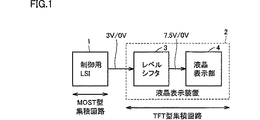••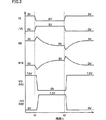•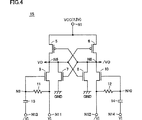•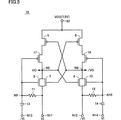•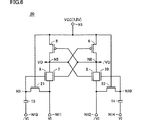•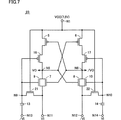••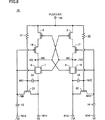•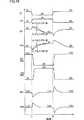•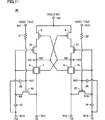•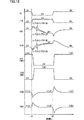•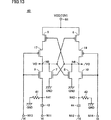•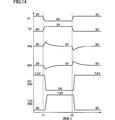•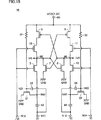•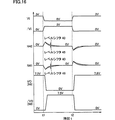•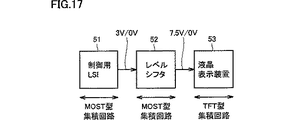•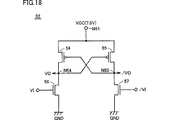•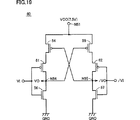## Classifications

• HELECTRICITY
• H03BASIC ELECTRONIC CIRCUITRY
• H03KPULSE TECHNIQUE
• H03K3/00Circuits for generating electric pulses; Monostable, bistable or multistable circuits
• H03K3/02Generators characterised by the type of circuit or by the means used for producing pulses
• H03K3/353Generators characterised by the type of circuit or by the means used for producing pulses by the use, as active elements, of field-effect transistors with internal or external positive feedback
• H03K3/356Bistable circuits
• H03K3/356104Bistable circuits using complementary field-effect transistors
• H03K3/356113Bistable circuits using complementary field-effect transistors using additional transistors in the input circuit
• GPHYSICS
• G09EDUCATION; CRYPTOGRAPHY; DISPLAY; ADVERTISING; SEALS
• G09GARRANGEMENTS OR CIRCUITS FOR CONTROL OF INDICATING DEVICES USING STATIC MEANS TO PRESENT VARIABLE INFORMATION
• G09G2310/00Command of the display device
• G09G2310/02Addressing, scanning or driving the display screen or processing steps related thereto
• G09G2310/0264Details of driving circuits
• G09G2310/0289Details of voltage level shifters arranged for use in a driving circuit
• HELECTRICITY
• H03BASIC ELECTRONIC CIRCUITRY
• H03KPULSE TECHNIQUE
• H03K17/00Electronic switching or gating, i.e. not by contact-making and –breaking
• H03K17/06Modifications for ensuring a fully conducting state
• H03K17/063Modifications for ensuring a fully conducting state in field-effect transistor switches

## Description


BACKGROUND OF THE INVENTION
The present invention relates to an amplitude conversion circuit, and more particularly to an amplitude conversion circuit for converting the amplitude of a signal.

[Prior art]
FIG. 17 is a block diagram showing a configuration of a portion related to image display of a conventional mobile phone.

In FIG. 17, this mobile phone includes a control LSI 51 that is a MOST (MOS transistor) type integrated circuit, a level shifter 52 that is a MOST type integrated circuit, and a liquid crystal display device 53 that is a TFT (thin film transistor) type integrated circuit. .

The control LSI 51 generates a control signal for the liquid crystal display device 53. The “H” level of this control signal is 3V, and its “L” level is 0V. Although a large number of control signals are actually generated, the number of control signals is one here for the sake of simplicity. The level shifter 52 converts the logic level of the control signal from the control LSI 51 to generate an internal control signal. The “H” level of this internal control signal is 7.5V, and its “L” level is 0V. The liquid crystal display device 53 displays an image according to an internal control signal from the level shifter 52.

FIG. 18 is a circuit diagram showing a configuration of the level shifter 52. In FIG. 18, level shifter 52 includes P channel MOS transistors 54 and 55 and N channel MOS transistors 56 and 57. P channel MOS transistors 54 and 55 are connected between node N51 of power supply potential VCC (7.5 V) and output nodes N54 and N55, respectively, and their gates are connected to output nodes N55 and N54, respectively. N channel MOS transistors 56 and 57 are connected between output nodes N54 and N55 and a node of ground potential GND, respectively, and their gates receive input signals VI and / VI, respectively.

Now, the input signals VI and / VI are set to “L” level (0V) and “H” level (3V), respectively, and the output signals VO and / VO are respectively set to “H” level (7.5V) and “L” level. It is assumed that it is set to (0V). At this time, the MOS transistors 54 and 57 are turned on, and the MOS transistors 55 and 56 are turned off.

In this state, the input signal VI rises from the “L” level (0 V) to the “H” level (3 V) and the input signal / VI rises from the “H” level (3 V) to the “L” level (0 V). When lowered, N channel MOS transistor 56 is first rendered conductive and the potential of output node N54 is lowered. When the potential of output node N54 becomes lower than the potential obtained by subtracting the absolute value of the threshold voltage of P channel MOS transistor 55 from power supply potential VCC, P channel MOS transistor 55 begins to conduct and the potential of output node N55 increases. Begin to. When the potential of output node N55 begins to rise, the voltage between the source and gate of P channel MOS transistor 54 decreases, the conduction resistance value of P channel MOS transistor 54 increases, and the potential of output node N54 further decreases. Therefore, the circuit operates in a positive feedback manner, and output nodes VO and / VO become “L” level (0 V) and “H” level (7.5 V), respectively, and the level conversion operation is completed.

FIG. 19 shows another conventional level shifter 6.0FIG. Referring to FIG. 19, level shifter 60 is different from level shifter 52 of FIG. 18 in that P-channel MOS transistors 61 and 62 are added. P channel MOS transistor 61 is interposed between the drain of P channel MOS transistor 54 and output node N54, and has its gate receiving input signal VI. P channel MOS transistor 62 is interposed between the drain of P channel MOS transistor 55 and output node N55, and has its gate receiving input signal / VI.

In level shifter 60, when input signal VI rises from "L" level (0V) to "H" level (3V), P-channel MOS transistor 61 changes from the conductive state to the non-conductive state, and the node of power supply potential VCC Since the current flowing from N51 to the output node N54 is reduced, the potential of the output node N54 tends to decrease. As a result, P channel MOS transistor 55 becomes conductive and the potential of output node N55 is likely to rise, so that the operation margin becomes larger than that of level shifter 52 in FIG.

Thus, in conventional level shifters 52 and 60, N channel MOS transistor 56 is rendered conductive in response to input signal VI being raised from "L" level (0V) to "H" level (3V). It is the premise of operation. In order for N channel MOS transistor 56 to be conductive, the threshold potential of N channel MOS transistor 56 needs to be equal to or lower than the “H” level (3 V) of input signal VI.

In a general semiconductor LSI, it is easy to reduce the threshold voltage of a transistor to 3 V or less. However, a low-temperature polysilicon TFT included in a liquid crystal display device has a large threshold voltage variation, and the threshold of the TFT is low. It is difficult to make the value voltage 3 V or less. Therefore, as shown in FIG. 17, a level shifter 52 or 60 composed of a high breakdown voltage MOS transistor is provided between the control LSI 51 and the liquid crystal display device 53 to convert the logic level of the signal.

[Problems to be solved by the invention]
However, when such level shifters 52 and 60 are provided, the cost of the level shifters 52 and 60 is added to the system cost, resulting in an increase in system cost.

Therefore, a main object of the present invention is to provide an amplitude conversion circuit which operates normally even when the amplitude voltage of the input signal is lower than the threshold voltage of the input transistor.

[Means for solving the problems and effects]
The amplitude conversion circuit according to the present invention converts a first signal whose amplitude is a first voltage into a second signal whose second amplitude is a second voltage higher than the first voltage. . In this amplitude conversion circuit, both of the first electrodes receive the second voltage, and the second electrodes are supplied to the first and second output nodes for outputting the second signal and its complementary signal. First and second transistors of the first conductivity type, each connected and having their input electrodes connected to the second and first output nodes, respectively, and those first electrodes being first and second, respectively. Driven by the first signal and its complementary signal, and in response to the leading edge of the first signal, from the first voltage A third voltage is applied between the input electrode and the second electrode of the third transistor to cause the third transistor to conduct, and the third voltage is applied to the fourth signal in response to the trailing edge of the first signal. Transistor input electrode and second It is provided a drive circuit for turning the fourth transistor is given between the electrodes.

thisThe driving circuit includes a first resistance element connected between the input electrode and the second electrode of the third transistor, one electrode receiving a complementary signal of the first signal, and the other electrode being the third transistor. A first capacitor connected to the input electrode of the second transistor, a second resistance element connected between the input electrode and the second electrode of the fourth transistor, one electrode receiving the first signal, and the other And a second capacitor connected to the input electrode of the fourth transistor for providing the first signal and its complementary signal to the second electrode of the third and fourth transistors, respectively.Accordingly, in response to the leading edge or the trailing edge of the first signal, a third voltage higher than the first voltage, which is the amplitude voltage of the first signal, is applied to the input electrode of the third or fourth transistor and the second voltage. So that the first signal operates even when the amplitude voltage of the first signal is lower than the threshold voltage of the third and fourth transistors.The

Preferably, the first resistance element includes an input electrode and a second electrode of the third transistor.whileAnd a fifth transistor whose input electrode receives the fourth voltage. The second resistance element includes a sixth transistor that is connected between the input electrode and the second electrode of the fourth transistor, and whose input electrode receives the fourth voltage. In this case, the area occupied by the first and second resistance elements may be small.

Also preferably, the fifth and sixth transistors are of the second conductivity type, and the fourth voltage is equal to the second voltage. In this case, the number of voltage sources can be small.

Preferably, the first resistance element includes a fifth transistor connected between the input electrode and the second electrode of the third transistor. The second resistance element includes a sixth transistor connected between the input electrode and the second electrode of the fourth transistor. The driving circuit further increases the resistance value of the fifth transistor in a pulse manner in response to the leading edge of the first signal, and increases the resistance value of the sixth transistor in response to the trailing edge of the first signal. A pulse generation circuit for increasing the pulse is included. In this case, the potential drop of the input electrodes of the third and fourth transistors can be moderated.

Also preferably, the fifth and sixth transistors are of the second conductivity type. The pulse generation circuit includes a third resistance element connected between a node of a fourth voltage having the same polarity as the second voltage and an input electrode of the fifth transistor, and one of the electrodes generates a first signal. A third capacitor having the other electrode connected to the input electrode of the fifth transistor, and a fourth resistance element connected between the node of the fourth voltage and the input electrode of the sixth transistor; , One electrode receiving a complementary signal of the first signal and the other electrode including a fourth capacitor connected to the input electrode of the sixth transistor. In this case, the input electrode of the fifth or sixth transistor charged to the fourth voltage via the third or fourth resistance element is equivalent to the first voltage via the third or fourth capacitor. Step down.

Also preferably, the fourth voltage is equal to the second voltage. In this case, the number of voltage sources can be small.

Also preferably, the fifth and sixth transistors are of the first conductivity type. The pulse generation circuit includes a third resistance element connected between a node of a fourth voltage having a polarity opposite to that of the second voltage and an input electrode of the fifth transistor, and one electrode of the first signal Receiving a complementary signal, a fourth capacitor connected between the third capacitor having the other electrode connected to the input electrode of the fifth transistor and the node of the fourth voltage and the input electrode of the sixth transistor; The resistor element includes a fourth capacitor having one electrode receiving the first signal and the other electrode connected to the input electrode of the sixth transistor. In this case, the input electrode of the fifth or sixth transistor charged to the fourth voltage via the third or fourth resistance element is equivalent to the first voltage via the third or fourth capacitor. Boosted.

Preferably, the drive circuit is further connected between the first diode element connected between the second electrode and the input electrode of the third transistor, and between the second electrode and the input electrode of the fourth transistor. And a second diode element. In this case, the input electrode of the third or fourth transistor can be quickly charged to the first voltage.

AlsoOther amplitude conversion circuit according to the present inventionIsThe first signal whose amplitude is the first voltage is converted into a second signal whose second amplitude is higher than the first voltage. In this amplitude conversion circuit, both of the first electrodes receive the second voltage, and the second electrodes are supplied to the first and second output nodes for outputting the second signal and its complementary signal. First and second transistors of the first conductivity type, each connected and having their input electrodes connected to the second and first output nodes, respectively, and those first electrodes being first and second, respectively. Driven by the first signal and its complementary signal, and in response to the leading edge of the first signal, from the first voltage A third voltage is applied between the input electrode and the second electrode of the third transistor to cause the third transistor to conduct, and the third voltage is applied to the fourth signal in response to the trailing edge of the first signal. Transistor input electrode and second A drive circuit for turning the fourth transistor is given between the electrodes is provided. thisThe driving circuit includes a first resistance element connected between the second electrode of the third transistor and the node of the reference voltage, one electrode receiving the first signal, and the other electrode being the third electrode. A first capacitor connected to the second electrode of the transistor; a second resistance element connected between the second electrode of the fourth transistor and a node of the reference voltage; And a second capacitor whose other electrode is connected to the second electrode of the fourth transistor, the first signal and its complementary signal being respectively received by the fourth and third transistors. To the input electrode.Accordingly, in response to the leading edge or the trailing edge of the first signal, a third voltage higher than the first voltage, which is the amplitude voltage of the first signal, is applied to the input electrode of the third or fourth transistor and the second voltage. So that the first signal operates even when the amplitude voltage of the first signal is lower than the threshold voltage of the third and fourth transistors.The

Preferably, the first resistance element includes a fifth transistor connected between the second electrode of the third transistor and the node of the reference voltage. The second resistance element includes a sixth transistor connected between the second electrode of the second transistor and the node of the reference voltage. The driving circuit further increases the resistance value of the fifth transistor in a pulse manner in response to the leading edge of the first signal, and increases the resistance value of the sixth transistor in response to the trailing edge of the first signal. A pulse generation circuit for increasing the pulse is included. In this case, the voltage increase of the input electrodes of the third and fourth transistors can be moderated.

Also preferably, the fifth and sixth transistors are of the second conductivity type. The pulse generation circuit includes a third resistance element connected between a node of a fourth voltage having the same polarity as the second voltage and an input electrode of the fifth transistor, and one of the electrodes generates a first signal. A third capacitor having the other electrode connected to the input electrode of the fifth transistor, and a fourth resistance element connected between the node of the fourth voltage and the input electrode of the sixth transistor; , One electrode receiving a complementary signal of the first signal and the other electrode including a fourth capacitor connected to the input electrode of the sixth transistor. In this case, the input electrode of the fifth or sixth transistor charged to the fourth voltage via the third or fourth resistance element is equivalent to the first voltage via the third or fourth capacitor. Step down.

Also preferably, the fourth voltage is equal to the second voltage. In this case, the number of voltage sources can be small.

Preferably, a latch circuit is further provided for latching the potentials of the first and second output nodes. In this case, the potentials of the first and second output nodes can be held stably.

Also preferably, the latch circuit has the first electrodes connected to the first and second output nodes, respectively, the second electrodes respectively receiving the first signal and its complementary signal, and their inputs. The electrodes include fifth and sixth transistors of the second conductivity type connected to the second and first output nodes, respectively. In this case, the latch circuit can be easily configured.

Preferably, the latch circuit is connected between the first and second output nodes and the node of the reference voltage, respectively, and the input electrodes thereof are connected to the second and first output nodes, respectively. It includes fifth and sixth transistors of conductive type. In this case, the driving force of the first signal and its complementary signal can be small.

Further preferably, the first conductive type having a first conductivity type interposed between the second electrode of the first transistor and the first output node, the input electrode of which is connected to the input electrode of the third transistor. The fifth transistor, the second electrode of the second transistor, and the second output node are interposed between the input electrodes of the fourth transistor and the first conductivity type. And a sixth transistor. In this case, current flowing from the second voltage node to the first and second output nodes can be reduced, and current consumption can be reduced.

Preferably, the first to fourth transistors are thin film transistors. The present invention is particularly effective in this case.

DETAILED DESCRIPTION OF THE INVENTION
[Embodiment 1]
FIG. 1 is a block diagram showing a configuration of a portion related to image display of a mobile phone according to Embodiment 1 of the present invention.

In FIG. 1, the cellular phone includes a control LSI 1 that is a MOST type integrated circuit and a liquid crystal display device 2 that is a TFT type integrated circuit. The liquid crystal display device 2 includes a level shifter 3 and a liquid crystal display unit 4.

The control LSI 1 outputs a control signal for the liquid crystal display device 2. The “H” level of this control signal is 3V, and its “L” level is 0V. Although a large number of control signals are actually generated, the number of control signals is one here for the sake of simplicity. The level shifter 3 converts the logic level of the control signal from the control LSI 1 to generate an internal control signal. The “H” level of this internal control signal is 7.5V, and its “L” level is 0V. The liquid crystal display unit 4 displays an image according to the internal control signal from the level shifter 3.

FIG. 2 is a circuit diagram showing the configuration of the level shifter 3. In FIG. 2, the level shifter 3 includes P-type TFTs 5 and 6, N-type TFTs 7 to 10, resistance elements 11 and 12, and capacitors 13 and 14. P-type TFTs 5 and 6 are connected between a node N1 of power supply potential VCC (7.5 V) and output nodes N5 and N6, respectively, and their gates are connected to output nodes N6 and N5, respectively. The signals appearing at the output nodes N5 and N6 become the output signals VO and / VO of the level shifter 3, respectively. N-type TFT 7 is connected between output node N5 and input node N11, and its gate is connected to output node N6. N-type TFT 8 is connected between output node N6 and input node N12, and its gate is connected to output node N5. Input nodes N11 and N12 receive input signals VI and / VI, respectively. P-type TFTs 5 and 6 and N-type TFTs 7 and 8 constitute a latch circuit for latching the levels of output nodes N5 and N6.

N-type TFT 9 is connected between input node N11 and output node N5, and its gate is connected to node N9. N-type TFT 10 is connected between input node N11 and output node N6, and its gate is connected to node N10. Resistance element 11 is connected between nodes N9 and N11, and resistance element 12 is connected between nodes N10 and N12. Capacitor 13 is connected between input node N13 and node N9, and capacitor 14 is connected between input node N14 and node N10. Input nodes N13 and N14 receive input signals / VI and VI, respectively. Resistive element 11 and capacitor 13 constitute a booster circuit, and resistive element 12 and capacitor 14 constitute a booster circuit.

FIG. 3 is a time chart showing the operation of the level shifter 3 shown in FIG. Referring to FIG. 3, in the initial state, input signals VI and / VI are set to “H” level (3 V) and “L” level (0 V), respectively, and output signals VO and / VO are set to “H” level ( 7.5V) and “L” level (0V). At this time, the node N9 is set to 3V having the same potential as the input signal VI by the resistance element 11, and the node N10 is set to 0V having the same potential as the input signal / VI by the resistance element 12. Due to these potential relationships, the P-type TFT 5 and the N-type TFT 8 are turned on, and the other TFTs 6, 7, 9, and 10 are turned off. That is, the output node N5 receives the power supply potential VCC (7.5V) via the P-type TFT 5, and the output node N6 receives the potential (0V) of the input signal / VI via the N-type TFT 8.

Next, at a certain time t1, the input signal VI falls from the “H” level (3V) to the “L” level (0V) and the input signal / VI changes from the “L” level (0V) to the “H” level ( 3V), the potential change of the input signal / VI is transmitted to the node N9 via the capacitor 13, and the potential of the node N9 rises to a potential of 3V or higher. The potential increase at this time is substantially determined by the ratio between the capacitance value of the capacitor 13 and the capacitance value of the parasitic capacitance (not shown) of the node N9. When the capacitor 13 sets the capacitance value to a value sufficiently larger than the capacitance value of the parasitic capacitance of the node N9, the node N9 rises to nearly 6V, which is twice the amplitude voltage (3V) of the input signals VI and / VI.

On the other hand, input signal VI falls from “H” level (3 V) to “L” level (0 V) simultaneously with / VI, so that the charge at node N9 is discharged to node N11 via resistance element 11. Therefore, the potential of the node N9 rises from 3V and reaches a peak value, and then gradually decreases to 0V. Here, by appropriately setting the resistance value of the resistance element 11, the potential of the node N9 can be held at a predetermined potential of 3 V or more for a predetermined time. When the potential of the node N9 becomes a predetermined potential, the N-type TFT 9 becomes conductive, and the potential of the output node N5 decreases. When the potential of the output node N5 decreases, the P-type TFT 6 becomes conductive and the potential of the output node N6 increases. As a result, the P-type TFT 5 becomes non-conductive, the N-type TFT 7 becomes conductive, and the potential of the output node N5 rapidly decreases to the “L” level (0 V).

On the other hand, the potential change of the input signal VI from the “H” level (3V) to the “L” level (0V) is transmitted to the node N10 via the capacitor 14, and the potential of the node N10 decreases from 0V to near −3V. . However, since the N-type TFT 10 is already non-conductive, there is no influence on the circuit operation.

As a result, the output signal VO is lowered from the “H” level (7.5 V) to the “L” level (0 V) and the output signal / VO is changed from the “L” level (0 V) to the “H” level (7. 5V), and a logic level conversion from 3V to 7.5V has been performed.

The potentials of the nodes N9 and N10 are shifted to the levels of the input signals VI and / VI with the passage of time by the resistance elements 11 and 12, respectively. At time t2, the potentials of the nodes N9 and N10 are at the levels of the input signals VI and / VI, respectively. At time t2, input signal / VI rises from "L" level (0V) to "H" level (3V) and input signal VI rises from "H" level (3V) to "L" level (0V). When lowered, the circuit operates in a potential relationship opposite to that described above.

In the first embodiment, in response to the falling edge of the input signal VI, a voltage (about 6V) higher than the amplitude voltage (3V) of the input signal VI is generated and applied between the gate and source of the N-type TFT 9. Even when the amplitude voltage (3 V) of the input signal VI is lower than the threshold voltage of the N-type TFT 9, the level shifter 3 operates. Therefore, as shown in FIG. 1, the level shifter 3 and the liquid crystal display unit 4 can be made into one liquid crystal display device 2 (TFT type integrated circuit). Therefore, the number of parts can be reduced and the system cost can be reduced as compared with the conventional case where the level shifter 52 and the liquid crystal display device 53 need to be provided separately.

Although the power supply current flows transiently during the operation, after the levels of the output nodes N5 and N6 are determined, the TFTs 5, 8, and 10 or the TFTs 6, 7, and 9 become non-conductive, so the node of the power supply potential VCC No direct current flows from N1 to input nodes N11 to N14. Therefore, the power consumption of the circuit is extremely small.

Although the TFTs 5 to 10 are used in the first embodiment, a MOS transistor may be used instead of the TFT. In this case, the operation is performed even when the amplitudes of the input signals VI and / VI are smaller than the threshold voltage of the MOS transistor.

In the first embodiment, a TFT which is an insulated gate field effect transistor is used. Needless to say, other types of field effect transistors may be used.

Hereinafter, various modifications of the first embodiment will be described. In the level shifter 15 of FIG. 4, the sources of the N-type TFTs 7 and 8 are grounded. In this modified example, since the current of the N-type TFTs 7 and 8 is supplied to the line of the ground potential GND without flowing to the input nodes N11 and N12, the driving force of the input signals VI and / VI can be reduced.

The level shifter 16 in FIG. 5 is different from the level shifter 3 in FIG. 2 in that P-type TFTs 17 and 18 are added. The P-type TFT 17 is interposed between the drain of the P-type TFT 5 and the node N5, and its gate is connected to the node N9. The P-type TFT 18 is interposed between the drain of the P-type TFT 6 and the node N6, and its gate is connected to the node N10. In this modification, for example, when the input signal / VI is raised from the “L” level (0 V) to the “H” level (3 V) (see time t1 in FIG. 3), the P-type TFT 17 is changed from the conductive state. Since the current changes from the node N1 of the power supply potential VCC to the output node N5 is suppressed, the potential of the output node N5 is likely to decrease. As a result, the P-type TFT 6 is quickly turned on, and the potential of the node N6 is likely to rise. Further, as described above, since the P-type TFTs 17 and 18 are turned off, the current flowing from the node N1 of the power supply potential VCC to the output nodes N5 and N6 is suppressed, so that the power consumption can be reduced.

The level shifter 20 in FIG. 6 differs from the level shifter 3 in FIG. 2 in that the resistance elements 11 and 12 are replaced by N-type TFTs 21 and 22, respectively. N-type TFT 21 is connected between nodes N9 and N11, and has its gate receiving power supply potential VCC. N-type TFT 22 is connected between nodes N10 and N12, and has its gate receiving power supply potential VCC. Each of the N-type TFTs 21 and 22 functions as a resistance element equivalently. Since the resistance value per unit dimension is higher than the resistance elements 11 and 12 of FIG. 2, the occupation area as the resistance element is reduced. Each of the N-type TFTs 21 and 22 may be replaced with a P-type TFT. However, in this case, it is necessary to apply a negative voltage (−7.5 V) to the gate of the P-type TFT.

The level shifter 23 in FIG. 7 differs from the level shifter 16 in FIG. 5 in that the resistance elements 11 and 12 are replaced by N-type TFTs 21 and 22, respectively. Therefore, this modified example has the effects of both the modified example of FIG. 5 and the modified example of FIG.

The level shifter 25 of FIG. 8 is obtained by adding diode elements 26 and 27 to the level shifter 16 of FIG. The diode element 26 is connected between the nodes N11 and N9, and the diode element27 is connected between nodes N12 and N14. The diode element 26 accelerates the rise of the node N9 to the “H” level (3V) when the input signal VI is raised from the “L” level (0V) to the “H” level (3V) (FIG. 3). reference). As a result, when the input signal / VI is subsequently raised from the “L” level (0 V) to the “H” level (3 V), the rise of the node N9 to the “H” level (3 V) becomes faster. The type TFT 9 conducts quickly. The diode element 27 has the same function as the diode element 26 for the N-type TFT 10. Therefore, in this modified example, the level change of the output signals VO and / VO with respect to the level change of the input signals VI and / VI becomes faster.

[Embodiment 2]
FIG. 9 is a circuit diagram showing the configuration of the level shifter 30 according to the second embodiment of the present invention, and is a diagram to be compared with FIG. Referring to FIG. 9, the level shifter 30 is different from the level shifter 23 of FIG. 7 in that resistance elements 31 and 32 and capacitors 33 and 34 are added. Resistance element 31 is inserted between node N1 of power supply potential VCC and the gate (node N21) of N-type TFT 21, and resistance element 32 is inserted between node N1 and the gate of N-type TFT 22 (node N22). It is. Capacitor 33 is connected between nodes N11 and N21, and capacitor 34Are connected between nodes N12 and N22.

FIG. 10 is a time chart showing the operation of the level shifter 30. In FIG. 10, in an initial state, input signals VI and / VI are set to “H” level (3 V) and “L” level (0 V), respectively, and output signals VO and / VO are set to “H” level (7.5 V, respectively). ) And “L” level (0 V). Since the nodes N21 and N22 receive the power supply potential VCC (7.5 V) via the resistance elements 31 and 32, respectively, the N-type TFTs 21 and 22 are conductive. Therefore, the node N9 is set to 3V having the same potential as the input signal VI, and the node N10 is set to 0V having the same potential as the input signal / VI. Due to these potential relationships, the P-type TFTs 5 and 16 and the N-type TFT 8 are conductive, and the other TFTs 6, 7, 9, 10, and 17 are non-conductive. That is, the output node N5 receives the power supply potential VCC (7.5 V) via the P-type TFTs 5 and 16, and the output node N6 receives the potential (0 V) of the input signal / VI via the N-type TFT 8.

Next, at a certain time t1, the input signal VI falls from the “H” level (3V) to the “L” level (0V) and the input signal / VI changes from the “L” level (0V) to the “H” level ( 3V), the potential change of the input signal / VI is transmitted to the node N9 via the capacitor 13, and the potential of the node N9 rises to a potential of 3V or higher. At the same time, the potential change of the input signal VI is transmitted to the node N29 via the capacitor 33, and the potential of the node N21 decreases by about 3V. When the potential of the node N21 decreases, power flows from the node N1 to the node N21 via the resistance element 31, and the node N21 returns to the power supply potential VCC (7.5 V). During the period when the node N21 is lower than 7.5V, the resistance value of the N-type TFT 21 is high.

At time t1, when the input signal VI falls from the “H” level (3V) to the “L” level (0V), the charge at the node N9 is discharged to the node N11 via the N-type TFT 21. Therefore, the potential of the node N9 rises from 3V and reaches a peak value, and then gradually decreases to 0V.

At this time, since the resistance value of the N-type TFT 21 becomes relatively high for a predetermined time, the level decrease of the node N1 becomes gentler than that of the level shifter 23 of FIG. Thereby, the conduction time of the N-type TFT 9 becomes longer, and the potential of the node N5 can be easily lowered.

On the other hand, on the node N10 side, at time t1, the input signal VI falls from the “H” level (3V) to the “L” level (0V) and the input signal / VI changes from the “L” level (0V) to “H”. When the voltage is raised to the level (3V), the potential change of input signal / VI is transmitted to node N10 via capacitor 14, and the potential of node N10 drops to a potential of 0V or less. At the same time, the potential change of the input signal / VI is transmitted to the node N22 via the capacitor 34, and the potential of the node N22 rises by about 3V. When the potential of node N22 rises, current flows from node N22 to node N21 via resistance element 32, and node N22 returns to power supply potential VCC (7.5 V). During the period when the potential of the node N22 is higher than 7.5V, the resistance value of the N-type TFT 22 is low.

Further, when the input signal / VI rises from the “L” level (0 V) to the “H” level (3 V) at time t1, a current flows from the node N12 to the node N10 via the N-type TFT 22. Accordingly, the potential of the node N10 decreases from 0V and reaches a peak value, and then gradually increases to 3V.

At this time, since the resistance value of the N-type TFT 22 becomes relatively low for a predetermined time, the level rise of the node N10 becomes faster than the level shifter 23 of FIG. Thereby, boosting of node N10 at the next time t2 can be easily performed.

As described above, the operation margin of the level shifter 30 is larger than the operation margin of the level shifter 23.

In the second embodiment, one electrode of resistance elements 31 and 32 is connected to node N1 of power supply potential VCC (7.5V), but connected to a node of positive power supply potential different from power supply potential VCC. Also good.

Each of the resistance elements 31 and 32 may be composed of an N-type TFT or a P-type TFT. A positive potential higher than the power supply potential VCC may be applied to the gate of the N-type TFT, and a potential lower than the power supply potential VCC may be applied to the gate of the P-type TFT. Further, the P-type TFTs 16 and 17 may be deleted.

[Embodiment 3]
FIG. 11 is a circuit diagram showing a configuration of the level shifter 35 according to the third embodiment of the present invention, and is a diagram to be compared with FIG. Referring to FIG. 11, the level shifter 35 is different from the level shifter 30 of FIG. 9 in that the N-type TFTs 21 and 22 are replaced with P-type TFTs 36 and 37. P-type TFT 36 is connected between nodes N9 and N11, and its gate is connected to node N21. P-type TFT 37 is connected between nodes N10 and N12, and its gate is connected to node N22.

Resistance element 31 is connected between node N21 and node N31 of negative power supply potential −VCC (−7.5 V). Resistance element 32 is connected between node N22 and node N32 of negative power supply potential −VCC (−7.5 V). Capacitor 33 is connected between nodes N13 and N21, and capacitor 34 is connected between nodes N14 and N22.

FIG. 12 is a time chart showing the operation of the level shifter 35. In FIG. 12, in the initial state, input signals VI and / VI are set to “H” level (3 V) and “L” level (0 V), respectively, and output signals VO and / VO are set to “H” level (7.5 V, respectively). ) And “L” level (0 V). Since the nodes N21 and N22 receive the negative power supply potential −VCC (−7.5 V) via the resistance elements 31 and 32, respectively, the P-type TFTs 36 and 37 are conductive. Therefore, the node N9 is set to 3V having the same potential as the input signal VI, and the node N10 is set to 0V having the same potential as the input signal / VI. Due to these potential relationships, the P-type TFTs 5 and 16 and the N-type TFT 8 are conductive, and the other TFTs 6, 7, 9, 10, and 17 are non-conductive. That is, the output node N5 receives the power supply potential VCC (7.5 V) via the P-type TFTs 5 and 16, and the output node N6 receives the potential (0 V) of the input signal / VI via the N-type TFT 8.

Next, at a certain time t1, the input signal VI falls from the “H” level (3V) to the “L” level (0V), and the input signal / VI becomes “L"From level (0V) to"HWhen raised to the level (3V), the potential change of input signal / VI is transmitted to node N9 through capacitor 13, and the potential of node N9 rises to a potential of 3V or higher. At the same time, the potential change of the input signal / VI is transmitted to the node N21 via the capacitor 33, and the potential of the node N21 rises by about 3V. When the potential of the node N21 rises, a current flows from the node N21 to the node N31 via the resistance element 31, and the node N21 returns to the negative power supply potential −VCC (−7.5 V). During the period when the potential of the node N21 is higher than −7.5V, the resistance value of the P-type TFT 36 is high.

At time t1, when the input signal VI falls from the “H” level (3V) to the “L” level (0V), the charge at the node N9 is discharged to the node N11 via the P-type TFT. Therefore, the potential of the node N9 rises from 3V and reaches a peak value, and then gradually decreases to 0V.

At this time, since the resistance value of the P-type TFT 36 becomes relatively high for a predetermined time, the level decrease of the node N9 becomes gentler than that of the level shifter 23 of FIG. Thereby, the conduction time of the N-type TFT 9 becomes longer, and the potential of the node N5 can be easily lowered.

On the other hand, on the node N10 side, at time t1, the input signal VI falls from the “H” level (3V) to the “L” level (0V) and the input signal / VI changes from the “L” level (0V) to “H”. When raised to the level (3V), the potential change of the input signal VI is transmitted to the node N10 via the capacitor 14, and the potential of the node N10 drops to a potential of 0V or less. At the same time, the potential change of the input signal VI is transmitted to the node N22 via the capacitor 34, and the potential of the node N22 decreases by about 3V. When the potential of the node N22 decreases, a current flows from the node N32 to the node N20 via the resistance element 32, and the node N22 returns to the negative power supply potential −VCC (−7.5 V). During the period when the potential of the node N22 is lower than −7.5V, the resistance value of the P-type TFT 37 is low.

Further, when the input signal / VI rises from the “L” level (0 V) to the “H” level (3 V) at time t1, a current flows from the node N12 to the node N10 via the P-type TFT 37. Accordingly, the potential of the node N10 decreases from 0V and reaches a peak value, and then gradually increases to 3V.

At this time, since the resistance value of the P-type TFT 37 becomes relatively low for a predetermined time, the level rise of the node N10 is faster than the level shifter 23 of FIG. Thereby, boosting of node N10 at the next time t2 can be easily performed.

As described above, the operation margin of the level shifter 35 is larger than the operation margin of the level shifter 23.

Each of the resistance elements 31 and 32 may be composed of an N-type TFT or a P-type TFT. A potential higher than the positive power supply potential VCC may be applied to the gate of the N-type TFT, and a negative potential lower than the negative power supply potential −VCC may be applied to the gate of the P-type TFT. Further, the P-type TFTs 16 and 17 may be deleted.

[Embodiment 4]
FIG. 13 is a circuit diagram showing a configuration of a level shifter 40 according to the fourth embodiment of the present invention, and is a diagram to be compared with FIG.

13, the level shifter 40 is different from the level shifter 16 of FIG. 5 in that the sources of the N-type TFTs 7 and 8 are both grounded, the resistance elements 11 and 12 and the capacitors 13 and 14 are resistance elements 41 and 42 and the capacitors 43 and 43, respectively. This is a point replaced with 44.

The capacitor 43 is connected between the input node N11 and the source of the N-type TFT 9 (node N41), and the capacitor 44 is connected between the input node N12 and the source of the N-type TFT 10 (node N42). Resistance elements 41 and 42 are respectively connected between nodes N41 and N42 and a line of ground potential GND. The input signal / VI is directly applied to the gates of the TFTs 9 and 17, and the input signal VI is directly applied to the gates of the TFTs 10 and 18.

FIG. 14 is a time chart showing the operation of the level shifter 40. Referring to FIG. 14, in the initial state, input signals VI and / VI are set to “H” level (3 V) and “L” level (0 V), respectively, and output signals VO and / VO are set to “H” level ( 7.5V) and “L” level (0V). The nodes N41 and N42 are set to the ground potential GND by the resistance elements 41 and 42. Due to these potential relationships, the P-type TFTs 5 and 17 and the N-type TFTs 8 and 10 are conductive, and the other TFTs 6, 7, 9, and 18 are non-conductive. That is, the output node N5 receives the power supply potential VCC (7.5 V) via the P-type TFTs 5 and 7, and the output node N6 receives the ground potential GND (0 V) via the N-type TFT 8.

Next, at a certain time t1, the input signal VI falls from the “H” level (3V) to the “L” level (0V) and the input signal / VI changes from the “L” level (0V) to the “H” level ( 3V), the potential change of the input signal VI is transmitted to the node N41 via the capacitor 43, and the node N41 falls to a potential equal to or lower than the ground potential GND (0V). The decrease in potential is determined by the ratio between the capacitance value of the capacitor 43 and the capacitance value of the parasitic capacitance (not shown) of the node N41. When the capacitance value of the capacitor 43 is set to a value sufficiently larger than the capacitance value of the parasitic capacitance, the potential of the node N41 decreases by the amplitude voltage of the input signal VI and decreases to −3V.

When the potential of the node N41 decreases to about −3V, a current flows into the node N41 from the ground potential GND line via the resistance element 41. Therefore, the potential of the node N41 decreases from 0V and reaches a peak value, and then gradually increases to 0V. Here, by appropriately setting the resistance value of the resistance element 41, the potential of the node N41 can be held at a predetermined potential of 0 V or less for a predetermined time.

When the node N41 becomes a predetermined potential, the voltage between the gate and the source of the N-type TFT 9 becomes 3V to 6V, the N-type TFT 9 becomes conductive, and the potential of the node N5 decreases. When the potential of the node N5 decreases, the P-type TFT 6 becomes conductive and the potential of the node N6 increases. As described above, the P-type TFT 5 becomes non-conductive and the N-type TFT 7 becomes conductive, and the potential of the output node N5 rapidly decreases to the “L” level (0 V).

On the other hand, the potential change of the input signal / VI from the “L” level (0V) to the “H” level (3V) is transmitted to the node N42 via the capacitor 44, and the potential of the node N42 increases from 0V to nearly 3V. . However, since the N-type TFT 10 is already non-conductive, there is no influence on the circuit operation.

As a result, the output signal VO is lowered from the “H” level (7.5 V) to the “L” level (0 V) and the output signal / VO is changed from the “L” level (0 V) to the “H” level (7. 5V), and a logic level conversion from 3V to 7.5V has been performed.

The potentials of the nodes N41 and N42 approach the ground potential GND with the passage of time by the resistance elements 41 and 42, respectively, and at the time t2, the potentials of the nodes N41 and N42 are substantially the ground potential GND. At time t2, input signal VI rises from "L" level (0V) to "H" level (3V) and input signal / VI rises from "H" level (3V) to "L" level (0V). When lowered, the circuit operates in a potential relationship opposite to that described above.

In the fourth embodiment, the same effect as in the first embodiment can be obtained.
As shown in FIG. 15, according to the second embodiment, resistance elements 41 and 42 are replaced with N-type TFTs 21 and 22, respectively, and between the gates (nodes N21 and N22) of N-type TFTs 21 and 22 and node N1. Resistance elements 31 and 32 may be connected to each other, and capacitors 33 and 34 may be connected between nodes N11 and N12 and nodes N21 and N22, respectively. As shown in FIG. 16, when the input signal VI falls from the “H” level (3V) to the “L” level (0V) at time t1, the potential of the node N21 decreases by about 3V, and the power supply potential is increased for a predetermined time. It becomes lower than VCC (7.5V). When the potential of the node N21 becomes lower than 7.5V, the resistance value of the N-type TFT 21 increases. Therefore, the level rise of the node N41 becomes more gradual than the level shifter 40 of FIG. 13, and the output node N5 is easily lowered to the “L” level. Further, when the input signal / VI rises from the “L” level (0 V) to the “H” level (3 V) at time t1, the potential of the node N22 rises by about 3 V, and the power supply potential VCC (7. 5V). When the potential of the node N21 becomes higher than 7.5V, the resistance value of the N-type TFT 22 becomes low. Therefore, the level decrease of node N42 becomes earlier than that of level shifter 40 in FIG. 13, and the step-down operation of node N42 at the next time t2 can be easily performed.

The embodiment disclosed this time should be considered as illustrative in all points and not restrictive. The scope of the present invention is defined by the terms of the claims, rather than the description above, and is intended to include any modifications within the scope and meaning equivalent to the terms of the claims.
[Brief description of the drawings]
FIG. 1 is a block diagram showing portions related to image display of a mobile phone according to Embodiment 1 of the present invention;
FIG. 2 is a circuit diagram showing a configuration of a level shifter shown in FIG.
FIG. 3 is a time chart showing the operation of the level shifter shown in FIG. 2;
FIG. 4 is a circuit diagram showing a modification of the first embodiment.
FIG. 5 is a circuit diagram showing another modification of the first embodiment.
FIG. 6 is a circuit diagram showing still another modification of the first embodiment.
FIG. 7 is a circuit diagram showing still another modification of the first embodiment.
FIG. 8 is a circuit diagram showing still another modified example of the first embodiment.
FIG. 9 is a circuit diagram showing a configuration of a level shifter according to a second embodiment of the present invention.
10 is a time chart showing the operation of the level shifter shown in FIG. 9;
FIG. 11 is a circuit diagram showing a configuration of a level shifter according to a third embodiment of the present invention.
12 is a time chart showing the operation of the level shifter shown in FIG. 11. FIG.
FIG. 13 is a circuit diagram showing a structure of a level shifter according to Embodiment 4 of the present invention.
14 is a time chart showing the operation of the level shifter shown in FIG.
FIG. 15 is a circuit diagram showing a modification of the fourth embodiment.
16 is a time chart showing the operation of the level shifter shown in FIG.
FIG. 17 is a block diagram showing portions related to image display of a conventional mobile phone.
FIG. 18 is a circuit diagram showing a configuration of the level shifter shown in FIG. 17;
FIG. 19 is a circuit diagram showing a configuration of another conventional level shifter.

## Claims (17)

1. An amplitude conversion circuit that converts a first signal whose amplitude is a first voltage into a second signal whose amplitude is a second voltage higher than the first voltage,
A first electrode thereof are both subjected to the second voltage, respectively connected to the first and second output nodes for their second electrodes to output said second signal and its complementary signal, First and second transistors of a first conductivity type with their input electrodes connected to the second and first output nodes, respectively;
The first electrodes are driven by the third and fourth transistors of the second conductivity type connected to the first and second output nodes, respectively, and the first signal and its complementary signal; Providing a third voltage higher than the first voltage in response to the leading edge of the first signal between the input electrode and the second electrode of the third transistor to cause the third transistor to conduct; A drive circuit for applying the third voltage between the input electrode and the second electrode of the fourth transistor in response to the trailing edge of the first signal to make the fourth transistor conductive ;
The drive circuit is
A first resistance element connected between an input electrode and a second electrode of the third transistor;
A first capacitor having one electrode receiving a complementary signal of the first signal and the other electrode connected to an input electrode of the third transistor;
A second resistance element connected between an input electrode and a second electrode of the fourth transistor; and
A second capacitor having one electrode receiving the first signal and the other electrode connected to the input electrode of the fourth transistor;
Ru given the first signal and the complementary signal to the respective second electrodes of said third and fourth transistors, the amplitude conversion circuit.
2. The first resistance element includes a fifth transistor connected between the input electrode and the second electrode of the third transistor, the input electrode receiving a fourth voltage;
The second resistor element, the fourth is connected between the input electrode and the second electrode of the transistor, a sixth transistor having an input electrode receiving said fourth voltage, according to claim 1 Amplitude conversion circuit.
3. The fifth and sixth transistors are of a second conductivity type;
The amplitude conversion circuit according to claim 2 , wherein the fourth voltage is equal to the second voltage.
4. The first resistance element includes a fifth transistor connected between an input electrode and a second electrode of the third transistor,
The second resistance element includes a sixth transistor connected between an input electrode and a second electrode of the fourth transistor,
The drive circuit further increases the resistance value of the fifth transistor in a pulse manner in response to the leading edge of the first signal, and the sixth circuit in response to the trailing edge of the first signal. 2. The amplitude conversion circuit according to claim 1 , further comprising a pulse generation circuit for increasing the resistance value of the transistor in a pulse manner.
5. The fifth and sixth transistors are of a second conductivity type;
The pulse generation circuit includes:
A third resistance element connected between a node of a fourth voltage having the same polarity as the second voltage and an input electrode of the fifth transistor;
A third capacitor having one electrode receiving the first signal and the other electrode connected to the input electrode of the fifth transistor;
A fourth resistance element connected between the node of the fourth voltage and an input electrode of the sixth transistor; and one electrode receives a complementary signal of the first signal, and the other electrode is The amplitude conversion circuit according to claim 4 , further comprising a fourth capacitor connected to the input electrode of the sixth transistor.
6. The amplitude conversion circuit according to claim 5 , wherein the fourth voltage is equal to the second voltage.
7. The fifth and sixth transistors are of a first conductivity type;
The pulse generation circuit includes:
A third resistance element connected between a node of a fourth voltage having a polarity opposite to that of the second voltage and an input electrode of the fifth transistor;
A third capacitor having one electrode receiving a complementary signal of the first signal and the other electrode connected to the input electrode of the fifth transistor;
A fourth resistance element connected between the node of the fourth voltage and an input electrode of the sixth transistor; and one electrode receives the first signal, and the other electrode is the sixth The amplitude conversion circuit according to claim 4 , further comprising a fourth capacitor connected to the input electrode of the transistor.
8. The drive circuit is
Furthermore, a first diode element connected between the second electrode and the input electrode of the third transistor, and a second diode element connected between the second electrode and the input electrode of the fourth transistor The amplitude conversion circuit according to claim 1 , comprising:
9. An amplitude conversion circuit that converts a first signal whose amplitude is a first voltage into a second signal whose amplitude is a second voltage higher than the first voltage,
Both of the first electrodes receive the second voltage, and the second electrodes are respectively connected to first and second output nodes for outputting the second signal and its complementary signal; First and second transistors of a first conductivity type with their input electrodes connected to the second and first output nodes, respectively;
Third and fourth transistors of a second conductivity type with their first electrodes respectively connected to the first and second output nodes; and
Driven by the first signal and its complementary signal and in response to a leading edge of the first signal, a third voltage higher than the first voltage is applied to an input electrode of the third transistor and a second voltage Providing the third transistor in conduction between the electrodes, and applying the third voltage between the input electrode and the second electrode of the fourth transistor in response to the trailing edge of the first signal, A drive circuit for conducting the fourth transistor;
The drive circuit is
A first resistance element connected between a second electrode of the third transistor and a node of a reference voltage;
A first capacitor having one electrode receiving the first signal and the other electrode connected to a second electrode of the third transistor;
A second resistance element connected between the second electrode of the fourth transistor and the node of the reference voltage; and one electrode receives a complementary signal of the first signal, and the other electrode is the includes a second Capacity data connected to the second electrode of the fourth transistor,
The first signal and the providing complementary signal to the input electrodes of the fourth and third transistors, the amplitude conversion circuit.
10. The first resistance element includes a fifth transistor connected between a second electrode of the third transistor and a node of the reference voltage,
The second resistance element includes a sixth transistor connected between a second electrode of the fourth transistor and a node of the reference voltage,
The drive circuit further increases the resistance value of the fifth transistor in a pulse manner in response to the leading edge of the first signal, and the sixth circuit in response to the trailing edge of the first signal. 10. The amplitude conversion circuit according to claim 9 , further comprising a pulse generation circuit for increasing the resistance value of the transistor in a pulse manner.
11. The fifth and sixth transistors are of a second conductivity type;
The pulse generation circuit includes:
A third resistance element connected between a node of a fourth voltage having the same polarity as the second voltage and an input electrode of the fifth transistor;
A third capacitor having one electrode receiving the first signal and the other electrode connected to the input electrode of the fifth transistor;
A fourth resistance element connected between the node of the fourth voltage and an input electrode of the sixth transistor; and one electrode receives a complementary signal of the first signal, and the other electrode is a fourth Capacity data connected to the input electrode of the sixth transistor, the amplitude conversion circuit of claim 1 0.
12. The fourth voltage is equal to the second voltage, the amplitude conversion circuit of claim 1 1.
13. The amplitude conversion circuit according to claim 1, further comprising a latch circuit for latching the potentials of the first and second output nodes.
14. The latch circuits have their first electrodes connected to the first and second output nodes, respectively, their second electrodes receiving the first signal and its complementary signal, respectively, and their input electrodes There including fifth and sixth transistors of the second conductivity type which is connected to each of the second and first output node, the amplitude conversion circuit according to claim 1 3.
15. The latch circuit is connected between the first and second output nodes and a reference voltage node, respectively, and has a second conductive circuit whose input electrodes are connected to the second and first output nodes, respectively. including fifth and sixth transistors form, the amplitude conversion circuit according to claim 1 3.
16. Furthermore, the first conductivity type second electrode is inserted between the second electrode of the first transistor and the first output node, and the input electrode of the first transistor is connected to the input electrode of the third transistor. 5, and a first conductive element that is interposed between the second electrode of the second transistor and the second output node, and whose input electrode is connected to the input electrode of the fourth transistor. The amplitude conversion circuit according to claim 1 or 9 , comprising a sixth transistor of the type.
17. The amplitude conversion circuit according to claim 1, wherein the first to fourth transistors are thin film transistors.
JP2003572178A 2002-02-26 2002-02-26 Amplitude conversion circuit Expired - Fee Related JP3927953B2 (en)

## Priority Applications (1)

Application Number Priority Date Filing Date Title
PCT/JP2002/001760 WO2003073617A1 (en) 2002-02-26 2002-02-26 Amplitude converting circuit

## Publications (2)

Publication Number Publication Date
JPWO2003073617A1 JPWO2003073617A1 (en) 2005-06-23
JP3927953B2 true JP3927953B2 (en) 2007-06-13

# Family

## Family Applications (1)

Application Number Title Priority Date Filing Date
JP2003572178A Expired - Fee Related JP3927953B2 (en) 2002-02-26 2002-02-26 Amplitude conversion circuit

## Country Status (6)

US (1) US6861889B2 (en)
JP (1) JP3927953B2 (en)
KR (1) KR100528858B1 (en)
CN (1) CN1258878C (en)
TW (1) TW546623B (en)
WO (1) WO2003073617A1 (en)

## Families Citing this family (13)

* Cited by examiner, † Cited by third party
Publication number Priority date Publication date Assignee Title
TWI297476B (en) * 2004-03-10 2008-06-01 Au Optronics Corp Voltage level shifter and sequential pulse generator
US7200053B2 (en) * 2004-09-01 2007-04-03 Micron Technology, Inc. Level shifter for low voltage operation
KR100590034B1 (en) * 2004-10-08 2006-06-14 삼성에스디아이 주식회사 Level shifter and display device using the same
KR20070111774A (en) * 2006-05-19 2007-11-22 삼성전자주식회사 Level shifter
JP5215534B2 (en) * 2006-05-19 2013-06-19 株式会社ジャパンディスプレイイースト Image display device
US7969808B2 (en) * 2007-07-20 2011-06-28 Samsung Electronics Co., Ltd. Memory cell structures, memory arrays, memory devices, memory controllers, and memory systems, and methods of manufacturing and operating the same
WO2009022275A1 (en) * 2007-08-13 2009-02-19 Nxp B.V. Level shifter circuit
KR20090116088A (en) * 2008-05-06 2009-11-11 삼성전자주식회사 A capacitor-less one transistor semiconductor memory device having improved data retention abilities and operation characteristics
KR20100070158A (en) * 2008-12-17 2010-06-25 삼성전자주식회사 Semiconductor memory device comprising capacitor-less dynamic memory cells, and method of operating the same
KR101442177B1 (en) * 2008-12-18 2014-09-18 삼성전자주식회사 Methods of fabricating a semiconductor device having a capacitor-less one transistor memory cell
KR20130096797A (en) * 2012-02-23 2013-09-02 에스케이하이닉스 주식회사 Voltage level converting circuit
US8723578B1 (en) * 2012-12-14 2014-05-13 Palo Alto Research Center Incorporated Pulse generator circuit
US10097183B2 (en) * 2016-03-30 2018-10-09 Texas Instruments Incorporated Level shifter and method of calibration

## Family Cites Families (11)

* Cited by examiner, † Cited by third party
Publication number Priority date Publication date Assignee Title
US3801831A (en) 1972-10-13 1974-04-02 Motorola Inc Voltage level shifting circuit
JPS5759690B2 (en) 1974-05-27 1982-12-16 Tokyo Shibaura Electric Co
JP3017133B2 (en) 1997-06-26 2000-03-06 日本電気アイシーマイコンシステム株式会社 Level shifter circuit
JP3697873B2 (en) 1997-12-05 2005-09-21 セイコーエプソン株式会社 Level shift circuit, signal driver and display device using the same, and semiconductor device
JP3481121B2 (en) * 1998-03-20 2003-12-22 松下電器産業株式会社 Level shift circuit
IT1304060B1 (en) * 1998-12-29 2001-03-07 St Microelectronics Srl level variator for circuitry at a voltage alimentazionemultipla
JP2001085989A (en) * 1999-09-16 2001-03-30 Matsushita Electric Ind Co Ltd Signal level conversion circuit and active matrix liquid crystal display device provided with the signal level conversion circuit
WO2001056159A1 (en) * 2000-01-27 2001-08-02 Hitachi, Ltd. Semiconductor device
US6359593B1 (en) * 2000-08-15 2002-03-19 Receptec Llc Non-radiating single slotline coupler
US20030169224A1 (en) * 2002-03-11 2003-09-11 Mitsubishi Denki Kabushiki Kaisha Amplitude conversion circuit for converting signal amplitude and semiconductor device using the amplitude conversion circuit
US6980194B2 (en) * 2002-03-11 2005-12-27 Mitsubishi Denki Kabushiki Kaisha Amplitude conversion circuit for converting signal amplitude

## Also Published As

Publication number Publication date
CN1488193A (en) 2004-04-07
KR100528858B1 (en) 2005-11-16
US6861889B2 (en) 2005-03-01
US20040041614A1 (en) 2004-03-04
WO2003073617A1 (en) 2003-09-04
JPWO2003073617A1 (en) 2005-06-23
KR20040000430A (en) 2004-01-03
TW546623B (en) 2003-08-11
CN1258878C (en) 2006-06-07

## Similar Documents

Publication Publication Date Title
JP6653247B2 (en) Semiconductor device
US9336897B2 (en) Shift register circuit
US6919743B2 (en) Drive circuit with low current consumption
DE4037206C2 (en) Supply voltage control circuit with the possibility of test-burn-in of an internal circuit
US7468624B2 (en) Step-down power supply
US7728652B2 (en) Semiconductor integrated circuit
US6753708B2 (en) Driver circuit connected to pulse shaping circuitry and method of operating same
US4217502A (en) Converter producing three output states
US7199623B2 (en) Method and apparatus for providing a power-on reset signal
JP2783044B2 (en) Boost circuit
US7005881B2 (en) Current sensing for power MOSFET operable in linear and saturated regions
DE4211644C2 (en) Circuit arrangement for generating a constant voltage
JP3286869B2 (en) Internal power supply potential generation circuit
JP2993461B2 (en) Drive circuit for liquid crystal display
US8159302B2 (en) Differential amplifier circuit
US7030682B2 (en) Voltage detection circuit and internal voltage generating circuit comprising it
US6373321B1 (en) CMOS semiconductor device
JP3752107B2 (en) Power-on reset circuit for integrated circuits
JP3832575B2 (en) Negative voltage output charge pump circuit
US20060202736A1 (en) Bootstrapped switch for sampling inputs with a signal range greater than supply voltage
KR930003926B1 (en) Semiconductor integrated circuit
US6750696B2 (en) Level conversion circuit converting logic level of signal
KR19990013822A (en) Level shift circuit
US7649384B2 (en) High-voltage tolerant output driver

## Legal Events

Date Code Title Description
A521 Written amendment

Free format text: JAPANESE INTERMEDIATE CODE: A523

Effective date: 20051107

A131 Notification of reasons for refusal

Free format text: JAPANESE INTERMEDIATE CODE: A131

Effective date: 20061219

A521 Written amendment

Free format text: JAPANESE INTERMEDIATE CODE: A523

Effective date: 20070201

TRDD Decision of grant or rejection written
A01 Written decision to grant a patent or to grant a registration (utility model)

Free format text: JAPANESE INTERMEDIATE CODE: A01

Effective date: 20070227

A61 First payment of annual fees (during grant procedure)

Free format text: JAPANESE INTERMEDIATE CODE: A61

Effective date: 20070305

R150 Certificate of patent or registration of utility model

Free format text: JAPANESE INTERMEDIATE CODE: R150

LAPS Cancellation because of no payment of annual fees